## What are Bundled Conductors?

We can often see the transmission lines where instead of a single conductor per phase multiple conductors per phase are being used. A metallic structure called spacers groups the conductors of a phase. These spaces help to maintain a constant distance between the conductors throughout their length, avoid clashing of conductors amongst themselves and also allowing them to be connected in parallel. Each phase can have two, three, or four conductors. The figures below show bundled conductors with spacers for the three configurations.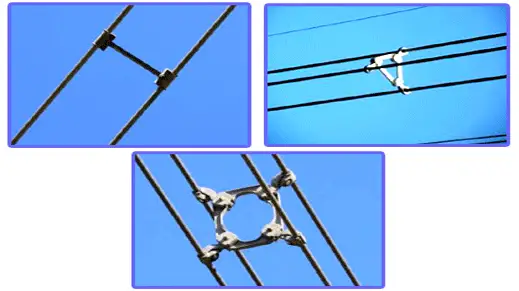Each conductor joined by the spacer belongs to the same phase, and we will have three such group of conductors in a single circuit transmission or six such groups in double circuit transmission.

We generally use such a configuration when a bulk power is being transmitted to long distances at very high voltage level.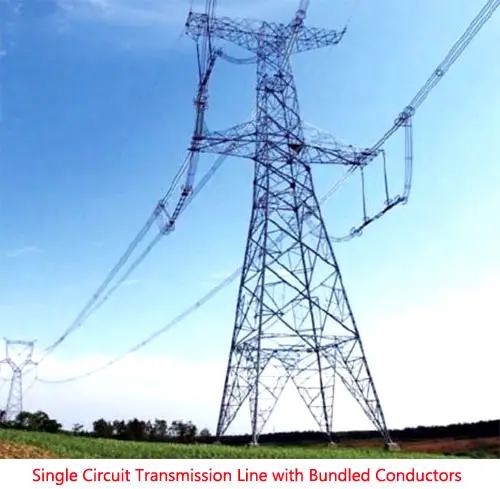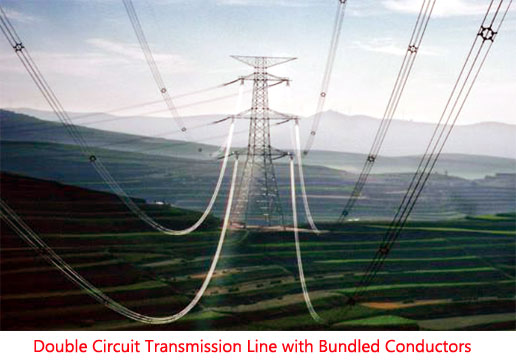Now we will see what the special advantages bundled conductors have over single conductor.

1. Bundling of conductors leads to reduction in line inductance.
We know that inductance of a line is given by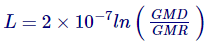Where, GMD = Geometric mean distance
For a single conductor of radius r
GMR = 0.7788r
For two conductor bundle as shown in figure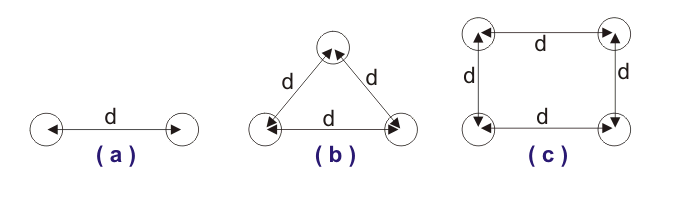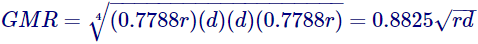For three conductor bundle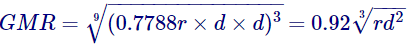For four conductor bundle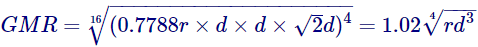Hence as we increase the number of conductors the GMR increases and hence L decrease. Now, there are many advantages of reduction in inductance of the line, such as-

• The maximum power transfer capability of the line increases as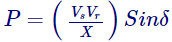Where X = wL …reactance of line

• The voltage regulation of the line is also increased as the reactance of the line is reduced.
2. On the similar argument for decrease in inductance of line, we can say that the capacitance of the line increases, as capacitance of line to neutral is given by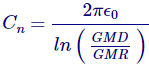Now since we have L decreased and C increased the net SIL of the line also increases automatically, and hence the power transfer capability too. Hence using bundled conductors is an effective way of increasing SIL, i.e. Surge Impedance Loading.
3. The most important advantage of bundled conductors is its ability to reduce corona discharge. When power is being transferred at very high voltages using a single conductor, the voltage gradient around it is high, and there is a high chance that the corona effect will occur – especially in bad weather conditions. However, using several conductors nearby instead of one conductor, forming a bundled conductor which leads to a reduction of voltage gradient and hence the possibility of corona formation.
The increase in critical corona voltage depends upon the following-

• Number of conductors in the group,
• Clearance between them, and
• The distance between the groups forming separate phases.

It has been found out that the optimum spacing between the conductors in a group is of the order of 8-10 times the diameter of each conductor, irrespective of the number of conductors in the bundle.

4. Reduction in the formation of corona discharge leads to less power loss and hence improved transmission efficiency of the line.
5. Reduction in communication line interference due to reduction in corona.
6. The ampacity i.e. the current carrying capacity of bundled conductors is much increased in comparison to single large conductor owing to reduced skin effect.
7. As the bundled conductors have more effective surface area exposed to air, it has better and efficient cooling and hence better performance compared to a single conductor.

Want To Learn Faster? 🎓
Get electrical articles delivered to your inbox every week.
No credit card required—it’s 100% free.